We're Open

100% Pass and No Plagiarism Guaranteed

# Answer: Element 011: MOD002616 Assessment

Element 011: MOD002616 Assessment

Question 1:

a)    The fixed steel bracket (shown in Figure Q1a) experiences applied forces F1 = 750 N, F2 = 900 N and F3 = 650 N.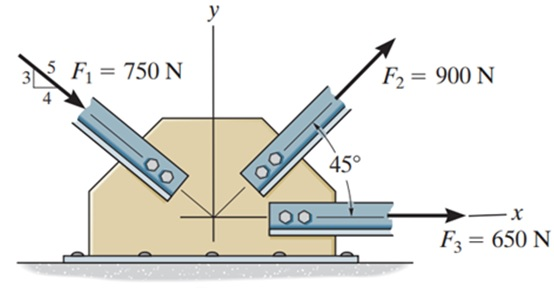Find:

i)     Resultant force in X direction.                                                                   (2 Marks)

ii)    Resultant force in Y direction                                                                    (2 Marks)

iii)   Resultant force and its direction measured anti-clockwise from the positive x- axis.                                                                                                                      (2 Marks)

b)   A rear wheel drive estate car has a prop-shaft connecting the gearbox to the differential, with prop-shaft details as follows:

• circular cross section
• outside diameter of 75 mm
• wall thickness1.0 mm
• length 1.1 m
• Maximum transmitted torque 1XY Nm (where X and Y are the last two digits of your SID, Student Identification Number)

Assuming that the Young’s modulus of the steel is 80 GPa, calculate:

i)     Maximum shear stress in the prop shaft.                                    (2 Marks)

ii)    Angular deflection of the prop-shaft.                                          (2 Marks)

c)     Provide a reflective commentary underpinning the KEY conceptual elements in solving this problem (maximum 100 words)                                      (6 Marks)

(Total Question Mark: 16)

Question 2:

a)    In the pin jointed truss (shown in Figure Q2a), point A is a pivot and point E is a roller pivot.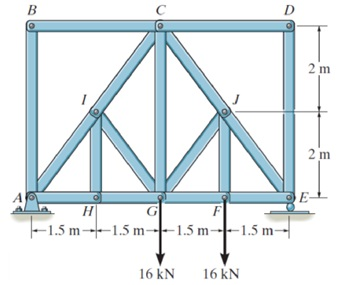FigureQ2a

i)     Determine the force in the members IC and CG of the truss, and state if they are in tension or compression                                                             (3 Marks)

ii)    Indicate any and/or all the zero-force members in the truss                (2 Marks)

b)   A thin wall, cylindrical steel boiler (shown in Figure Q2b) has a 1500 mm diameter and is subjected to an internal pressure of 2.5 N/mm2. If the thickness of the boiler plate is 2Y.40 mm (where Y is the last digit of your SID, Student Identification Number), determine:

i) The axial stress in the walls of the boiler                                                   (2 Marks)

ii) The hoop stress in the walls of the boiler                                                 (2 Marks)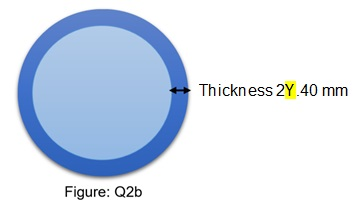c)     Provide a reflective commentary underpinning the KEY conceptual elements in solving this problem (maximum 100 words)                                                    (6 Marks)

(Total Question Mark: 15)

Question 3

The rotor shown in Figure Q3, has four masses m1, m2, m3 and m4 are 200 kg, 300 kg, 240 kg and 260 kg respectively. The corresponding radii of rotation of the masses are 0.2 m, 0.15 m, 0.25 m, and 0.3 m respectively, and the angles between successive masses are 45°, 75° and 135°

a)    At a radius of rotation of 0.2 m, Find:

i)          The weight of the balancing mass m to be attached to give dynamic balance.                                                                                     (8 Marks)

ii)         The position of the balancing mass m found in part (i) calculated anticlockwise from the positive x-axis.                                                (2 Marks)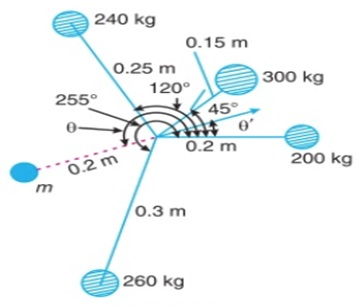Figure: Q3

b)   Provide a reflective commentary underpinning the KEY conceptual elements in solving this problem (maximum 100 words)                                         (6 Marks)

(Total Question Mark: 16)

Question 4

Consider the I-beam as shown in Figure Q4.

a)   Calculate the second moment of area of the I-beam

(2 Marks)

b)   Draw the shear force and bending moment diagram along the I-beam

(4 Marks)

c)   Calculate the maximum bending stress in the I-beam

(3 Marks)

d)   Calculate the maximum defection of the I-beam

(3 Marks)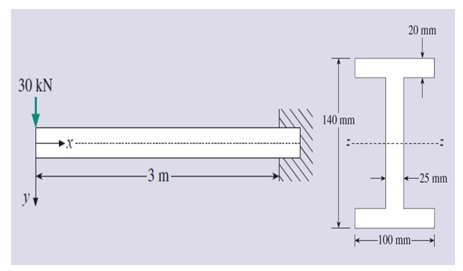Figure Q4

e)    Provide a reflective commentary underpinning the KEY conceptual elements in solving this problem (maximum 100 words)(6 Marks)

(Total Question Mark: 18)

Question 5

The Frame in the image below (Figure Q5) has a rigid joint at B, and joint C is an internal hinge of the frame.

a)

i) Determine the support reactions at point A and D. (4 Marks)

ii) Construct axial force, shear force and bending moment diagram for the frame stating relevant forces and moments.

(9 Marks)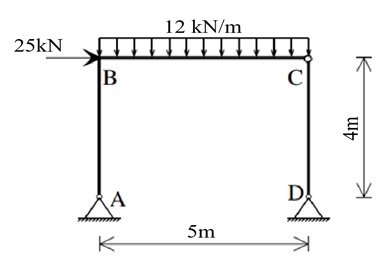Figure: Q5

b)   Provide a reflective commentary underpinning the KEY conceptual elements in solving this problem (maximum 100 words)

(6 Marks)

(Total Question Mark: 19)

Question 6

If the mass of the suspended flowerpot (shown in Figure Q6) is 3Y Kg (where Y is the last digit of your SID, Student Identification Number), and x = 1.2 m and z = 1.8 m.

a)    Determine the tension developed in wire CA, DA and AB for equilibrium. (10 Marks)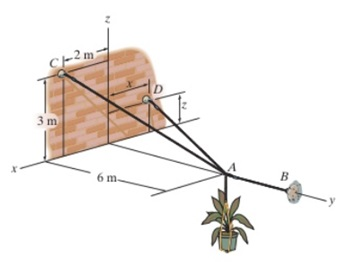Figure: Q6

b)         Provide a reflective commentary underpinning the KEY conceptual elements in solving this problem (maximum 100 words)

(6 Marks)

(Total Question Mark: 16)

100% Plagiarism Free & Custom Written,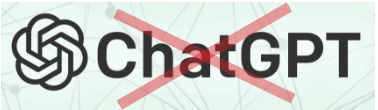The services provided by Assignment Experts UK are 100% original and custom written. We never use any paraphrasing tool, any software to generate content for e.g. Chat GPT and all other content writing tools. We ensure that the work produced by our writers is self-written and 100% plagiarism-free.

International House, 12 Constance Street, London, United Kingdom,
E16 2DQ

## STILL NOT CONVINCED?

We've produced some samples of what you can expect from our Academic Writing Service - these are created by our writers to show you the kind of high-quality work you'll receive. Take a look for yourself!